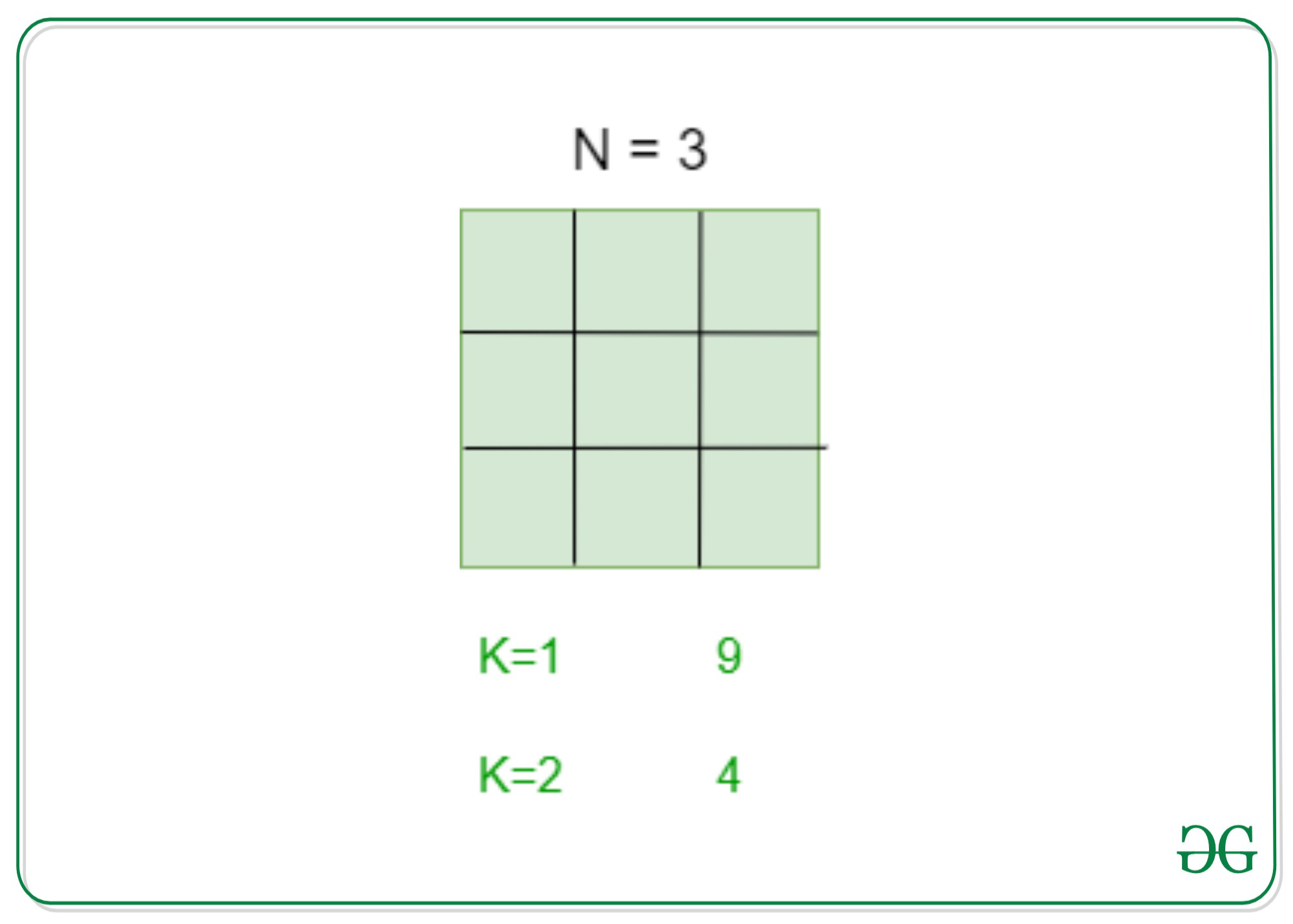# Count squares of size K inscribed in a square of size N

• Difficulty Level : Medium
• Last Updated : 16 Apr, 2021

Given two integers N and K, the task is to find the number of squares of size K that is inscribed in a square of size N.Attention reader! Don’t stop learning now. Get hold of all the important DSA concepts with the DSA Self Paced Course at a student-friendly price and become industry ready.  To complete your preparation from learning a language to DS Algo and many more,  please refer Complete Interview Preparation Course.

In case you wish to attend live classes with experts, please refer DSA Live Classes for Working Professionals and Competitive Programming Live for Students.

Examples:

Input: N = 4, K = 2
Output: 9
Explanation:
There are 9 squares of size 2 inscribed in a square of size 4.

Input: N = 5, K = 3
Output: 9
Explanation:
There are 9 squares of size 3 inscribed in a square of size 5.

Approach: The key observation to solve the problem is that the total number of squares in a square of size N is (N * (N + 1)* (2*N + 1)) / 6. Therefore, the total number of squares of size K possible from a square of size N are:Below is the implementation of the above approach:

## C++

 `// C++ implementation of the``// above approach` `#include ``using` `namespace` `std;` `// Function to calculate the number``// of squares of size K in a square``// of size N``int` `No_of_squares(``int` `N, ``int` `K)``{``    ``// Stores the number of squares``    ``int` `no_of_squares = 0;` `    ``no_of_squares``        ``= (N - K + 1) * (N - K + 1);` `    ``return` `no_of_squares;``}` `// Driver Code``int` `main()``{``    ``// Size of the``    ``// bigger square``    ``int` `N = 5;` `    ``// Size of``    ``// smaller square``    ``int` `K = 3;``    ``cout << No_of_squares(N, K);``    ``return` `0;``}`

## Java

 `// Java implementation of the``// above approach``import` `java.util.*;``class` `GFG{` `// Function to calculate the``// number of squares of size``// K in a square of size N``static` `int` `No_of_squares(``int` `N,``                         ``int` `K)``{``  ``// Stores the number``  ``// of squares``  ``int` `no_of_squares = ``0``;` `  ``no_of_squares = (N - K + ``1``) *``                  ``(N - K + ``1``);` `  ``return` `no_of_squares;``}` `// Driver Code``public` `static` `void` `main(String[] args)``{``  ``// Size of the``  ``// bigger square``  ``int` `N = ``5``;` `  ``// Size of``  ``// smaller square``  ``int` `K = ``3``;``  ``System.out.print(No_of_squares(N, K));``}``}` `// This code is contributed by Princi Singh`

## Python3

 `# Python3 implementation of the``# above approach` `# Function to calculate the``# number of squares of size``# K in a square of size N``def` `No_of_squares(N, K):``  ` `    ``# Stores the number``    ``# of squares``    ``no_of_squares ``=` `0``;``    ``no_of_squares ``=` `(N ``-` `K ``+` `1``) ``*` `(N ``-` `K ``+` `1``);``    ``return` `no_of_squares;` `# Driver Code``if` `__name__ ``=``=` `'__main__'``:``  ` `    ``# Size of the``    ``# bigger square``    ``N ``=` `5``;` `    ``# Size of``    ``# smaller square``    ``K ``=` `3``;``    ``print``(No_of_squares(N, K));` `# This code is contributed by 29AjayKumar`

## C#

 `// C# implementation of the``// above approach``using` `System;` `class` `GFG{` `// Function to calculate the``// number of squares of size``// K in a square of size N``static` `int` `No_of_squares(``int` `N, ``int` `K)``{``    ` `    ``// Stores the number``    ``// of squares``    ``int` `no_of_squares = 0;``    ` `    ``no_of_squares = (N - K + 1) *``                    ``(N - K + 1);``    ` `    ``return` `no_of_squares;``}` `// Driver Code``public` `static` `void` `Main(String[] args)``{``    ` `    ``// Size of the``    ``// bigger square``    ``int` `N = 5;``    ` `    ``// Size of``    ``// smaller square``    ``int` `K = 3;``    ` `    ``Console.Write(No_of_squares(N, K));``}``}` `// This code is contributed by Amit Katiyar`

## Javascript

 ``
Output:
`9`

Time Complexity: O(1)
Auxiliary Space: O(1)

My Personal Notes arrow_drop_up